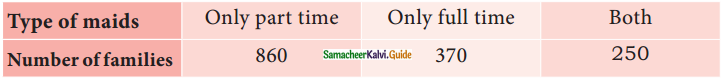Students can download Maths Chapter 9 Probability Ex 9.2 Questions and Answers, Notes, Samacheer Kalvi 9th Maths Guide Pdf helps you to revise the complete Tamilnadu State Board New Syllabus, helps students complete homework assignments and to score high marks in board exams.

## Tamilnadu Samacheer Kalvi 9th Maths Solutions Chapter 9 Probability Ex 9.2

Question 1.
A company manufactures 10000 Laptops in 6 months. In that 25 of them are found to be defective. When you choose one Laptop from the manufactured, what is the probability that selected Laptop is a good one?
Solution:
Total number of laptops = 10000
∴ n(S) = 10000
Number of good laptops = 10000 – 25 = 9975
Let E be the event of getting good laptops
n(E) = 9975
P(E) = $$\frac{n(E)}{n(S)}$$
= $$\frac{9975}{10000}$$
= $$\frac{399}{400}$$ (or)
= 0.9975Question 2.
In a survey of 400 youngsters aged 16-20 years, it was found that 191 have their voter ID card. If a youngster is selected at random, find the probability that the youngster does not have their voter ID card.
Solution:
Here n(S) = 400
Number of persons having voter ID = 191
Number of persons does not have their voter ID
= 400 – 191
= 209
n(E) = 209
P(E) = $$\frac{n(E)}{n(S)}$$
= $$\frac{209}{400}$$
∴ The required probability is $$\frac{209}{400}$$Question 3.
The probability of guessing the correct answer to a certain question is $$\frac{x}{3}$$. If the probability of not guessing the correct answer is $$\frac{x}{5}$$, then find the value of x.
Solution:
Probability of guessing the correct answer = $$\frac{x}{3}$$
P(E) = $$\frac{x}{3}$$
Probability of not guessing the correct answer = $$\frac{x}{5}$$
P(E)’ = $$\frac{x}{5}$$
But P(E) + P(E)’ = 1
$$\frac{x}{3}$$ + $$\frac{x}{5}$$ = 1
$$\frac{5x+3x}{15}$$ = 1 ⇒ $$\frac{8x}{15}$$ = 1
8x = 15
x = $$\frac{15}{8}$$
∴ The value of x = $$\frac{15}{8}$$Question 4.
If a probability of a player winning a particular tennis match is 0.72. What is the probability of the player loosing the match?
Solution:
Probability of a player winning a tennis match = 0.72
P(E) = 0.72
Probability of a player loosing the match be P(E)’
But P(E) + P(E)’ = 1
0.72 + P(E)’ = 1
P(E)’ = 1 – 0.72
= 0.28
Probability of the player loosing the match = 0.28Question 5.
1500 families were surveyed and following data was recorded about their maids at homesA family selected at random. Find the probability that the family selected has
(i) Both types of maids
(ii) Part time maids
(iii) No maids
Solution:
Total number of families surveyed = 1500
n(S) = 1500
Number of families used maids = 860 + 370 + 250
= 1480
Number of families not using any maids = 1500 – 1480
= 20

(i) Let E1 be the event of getting families use both types of maids
n(E1) = 250
p(E1) = $$\frac{n(E_{1})}{n(S)}$$
= $$\frac{250}{1500}$$
= $$\frac{25}{150}$$
= $$\frac{1}{6}$$
Probability of getting both types of maids = $$\frac{1}{6}$$(ii) Let E2 be the event of getting families use part time maids
n(E2) = 860
p(E2) = $$\frac{n(E_{2})}{n(S)}$$
= $$\frac{860}{1500}$$
= $$\frac{43}{75}$$
Probability of getting part time maids = $$\frac{43}{75}$$

(iii) Let E3 be the event of getting family use no maids
n(E3) = 20
p(E3) = $$\frac{n(E_{3})}{n(S)}$$
= $$\frac{20}{1500}$$
= $$\frac{2}{150}$$
= $$\frac{1}{75}$$
Probability of getting no maids = $$\frac{1}{75}$$